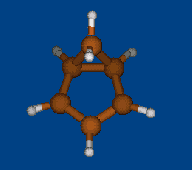Новосибирский институт органической химии им. Н.Н. Ворожцова СО РАН

Лаборатория изучения механизмов органических реакций

# xyz2inp

### Usage: `xyz2inp [-option[=string]] file.xyz [file1.xyz ...]`

#### Dependencies: `perl, [symmetry, babel].`

```Prepare input files for GAMESS from xyz geometry.

-run=str  Default optimize. For -run=SADPOINT sets hess=calc in \$statpt.
For run=IRC puts \$irc group with evaluated IRC step.
-dft=str  Functional for DFT. -dft is -dft=B3LYP
-mp=N     MPLEVL. -mp is -mp=2
-basis='str'  Default 6-31G*. Pople's N21/N31/N311 is parsed.
F.e. -basis='6-311++G**'
If -basis=priSET, then it is Priroda basis SET from
basis.in (after each atom in\$DATA group).
f.e. -basis=priL1
-mult=N    Multiplicity. scftyp=UHF for -mult=2
-charge=N  Charge. If dosn't specified, then calculated (0 or 1).
-zmt       Transform xyz to z-matrice. Babel needs. Force -nodlc.
-nodlc     Force no DLC. Default for -zmt.
-nosymm    Force C1 symmetry.

```
Последняя модификация: Thu Sep 1 14:27:56 2011